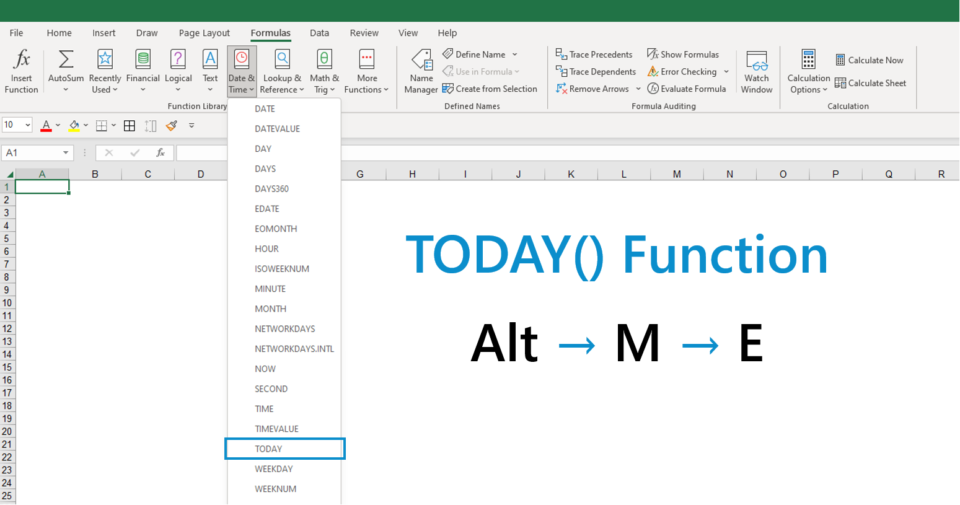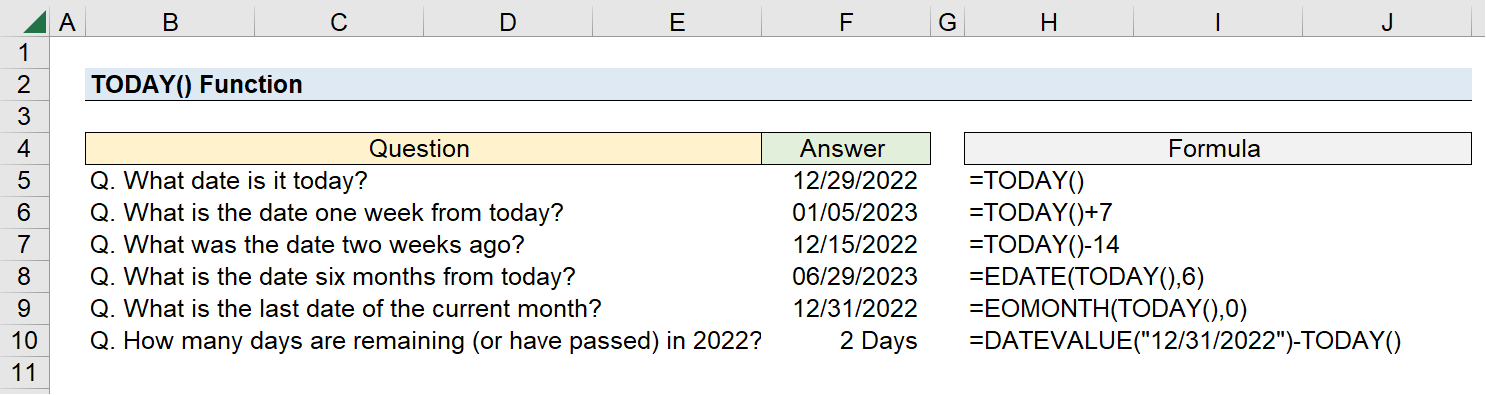Welcome to Wall Street Prep! Use code at checkout for 15% off.# TODAY Function

Guide to Understanding the TODAY() Function in Excel## How to Use TODAY Function in Excel

The Excel TODAY() function can be entered into a cell to return the current date. The output of the TODAY function date—the present date—is continuously updated to the date on which the file was most recently opened.

Since the function is automated in that sense, one scenario where the function is often utilized is for printing spreadsheets or exporting the file into PDF format for distribution.

The following are some of the more common use cases of the TODAY() function.

• Time-Stamping: The date on which the file was last updated is stated on the static version of the file. Thus, the TODAY function operates essentially like a timestamp, which can be useful for internal documentation purposes.
• Error-Proofing: For instance, suppose a financial model was updated, yet the exported data (e.g. a football field valuation chart) was mistakenly not updated. The date being on the graph increases the likelihood of someone catching a potential date-based error.
• A/R Invoicing: In another real-world example, conditional formatting can be used on a data set consisting of invoices, where the cells containing dates before the current date (i.e. less than) are highlighted. The highlighted cells signify that those invoices are overdue payments, in which a follow-up with the customer would be warranted. Because the date is updated automatically, the process of checking for past-due payments becomes more convenient.

## TODAY Function Shortcut: Insert Today’s Date into Excel

The “TODAY” function is located in the drop-down list from the “Date & Time” section within the “Formulas” tab.

The following keys can also be pressed to open the function.

TODAY() Keyboard Shortcut Alt + M + E

## Excel TODAY() Function Syntax and Format

The syntax for the Excel TODAY() function is as follows.

=TODAY()

As evident by the formula above, the function requires no arguments.

But before using the function, ensure the cell(s) are all properly formatted as dates, otherwise the returned value will be a numerical string.

If you need to keep the date static, as opposed to the date automatically updating, copy the cell with today’s date and use the paste special function to paste only the value.

While the TODAY function is rather straightforward, the following table contains some variations where the function can be used:

Formula Description
=TODAY()+10
• The returned value is the date ten days after the current date.
=TODAY()-10
• The returned value is the date ten days before the current date.
=DATEVALUE(“mm/dd/yyyy”)-TODAY()
• The returned value is the number of days in between a specified date and the current date.
=DAY(TODAY())
• The returned value is the day portion of the current date, e.g. “10” if the date is January 10, 2023.
=MONTH(TODAY())
• The returned value is the month portion of the current date, e.g. “1” if the date is January 10, 2023.
=YEAR(TODAY())
• The returned value is the year portion of the current date, e.g. “2023” if the date is January 10, 2023.
=EDATE(TODAY(),10)
• The returned value is the date ten months from the present date.
=EOMONTH(TODAY(),0)
• The returned value is the last date of the current month.

## TODAY Function Calculator – Excel Model Template

We’ll now move on to a modeling exercise, which you can access by filling out the form below.Submitting...

## Excel TODAY() Function Exercise Example

• Q. What date is it today?
• Q. What is the date one week from today?
• Q. What was the date two weeks ago?
• Q. What is the date six months from today?
• Q. What is the last date of the current month?
• Q. How many days are remaining (or have passed) in 2022?

To start, we’ll enter “TODAY()” into a cell to determine the current date, which comes out as 12/29/2022.

Because the dates automatically update, the answers displayed on the file will change each passing day.

The following formulas will be used to answer the above questions:

=TODAY()
=TODAY()+7
=TODAY()-14
=EDATE(TODAY(),6)
=EOMONTH(TODAY(),0)
=DATEVALUE(“12/31/2022”)-TODAY()

Using the TODAY() function, we arrive at the following answers (based upon an assumed current date of 12/29/2022):

• A. 12/29/2022
• A. 01/05/2023
• A. 12/15/2022
• A. 06/29/2023
• A. 12/31/2022
• A. 2 DaysTurbo-charge your time in Excel Used at top investment banks, Wall Street Prep's Excel Crash Course will turn you into an advanced Power User and set you apart from your peers.